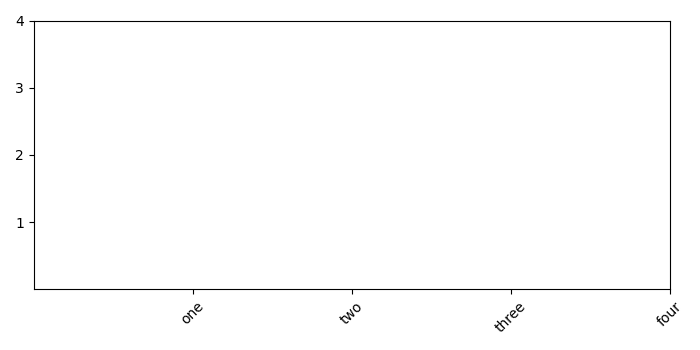# How to rotate xticklabels in Matplotlib so that the spacing between each xticklabel is equal?

MatplotlibServer Side ProgrammingProgramming

#### Python Data Science basics with Numpy, Pandas and Matplotlib

Most Popular

63 Lectures 6 hours

#### Data Visualization using MatPlotLib & Seaborn

11 Lectures 4 hours

#### MatPlotLib with Python

9 Lectures 2.5 hours

To rotate xticklabels in matplotlib to make equal spacing between two xticklabels, we can take the following steps −

• Make a list of numbers from 1 to 4.

• Using subplot(), sdd a subplot to the current figure.

• Add xticks and yticks on the current subplot (using step 1).

• Set xtick labels by passing a list and to make label rotation (= 45).

• To display the figure, use show() method.

## Example

import matplotlib.pyplot as plt
plt.rcParams["figure.figsize"] = [7.00, 3.50]
plt.rcParams["figure.autolayout"] = True
x = [1, 2, 3, 4]
ax1 = plt.subplot()
ax1.set_xticks(x)
ax1.set_yticks(x)
ax1.set_xticklabels(["one", "two", "three", "four"], rotation=45)
plt.show()

## Output